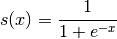GeeksforGeeks App
Open AppBrowser
Continue

Theano is a Python library that allows us to evaluate mathematical operations including multi-dimensional arrays so efficiently. It is mostly used in building Deep Learning Projects. It works a way more faster on Graphics Processing Unit (GPU) rather than on CPU. Theano attains high speeds that gives a tough competition to C implementations for problems involving large amounts of data. It can take advantage of GPUs which makes it perform better than C on a CPU by considerable orders of magnitude under some certain circumstances.
It knows how to take structures and convert them into very efficient code that uses numpy and some native libraries. It is mainly designed to handle the types of computation required for large neural network algorithms used in Deep Learning. That is why, it is a very popular library in the field of Deep Learning.

How to install Theano :

`pip install theano`

Several of the symbols we will need to use are in the tensor subpackage of Theano. We often import such packages with a handy name, let’s say, T.

```from theano import *
import theano.tensor as T```

Why Theano Python Library :
Theano is a sort of hybrid between numpy and sympy, an attempt is made to combine the two into one powerful library. Some advantages of theano are as follows:

• Stability Optimization: Theano can find out some unstable expressions and can use more stable means to evaluate them
• Execution Speed Optimization: As mentioned earlier, theano can make use of recent GPUs and execute parts of expressions in your CPU or GPU, making it much faster than Python
• Symbolic Differentiation: Theano is smart enough to automatically create symbolic graphs for computing gradients

Basics of Theano :
Theano is a Python library that allows you to define, optimize, and evaluate mathematical expressions involving multi-dimensional arrays efficiently.Some Theano implementations are as follows.

Subtracting two scalars :

## Python

 `# Python program showing``# subtraction of two scalars` `import` `theano``from` `theano ``import` `tensor` `# Declaring variables``a ``=` `tensor.dscalar()``b ``=` `tensor.dscalar()` `# Subtracting``res ``=` `a ``-` `b``# Converting it to a callable object``# so that it takes matrix as parameters``func ``=` `theano.function([a, b], res)` `# Calling function``assert` `20.0` `=``=` `func(``30.5``, ``10.5``)`

It will not provide any output as the assertion of two numbers matches the number given, hence it results into a true value.

## Python

 `# Python program showing``# addition of two scalars` `# Addition of two scalars``import` `numpy``import` `theano.tensor as T``from` `theano ``import` `function` `# Declaring two variables``x ``=` `T.dscalar(``'x'``)``y ``=` `T.dscalar(``'y'``)` `# Summing up the two numbers``z ``=` `x ``+` `y` `# Converting it to a callable object``# so that it takes matrix as parameters``f ``=` `function([x, y], z)``f(``5``, ``7``)`

Output:

`array(12.0)`

## Python

 `# Python program showing``# addition of two matrices` `# Adding two matrices``import` `numpy``import` `theano.tensor as T``from` `theano ``import` `function``x ``=` `T.dmatrix(``'x'``)``y ``=` `T.dmatrix(``'y'``)``z ``=` `x ``+` `y``f ``=` `function([x, y], z)` `f([[``30``, ``50``], [``2``, ``3``]], [[``60``, ``70``], [``3``, ``4``]])`

Output:

```array([[ 90.,  120.],
[ 5.,  7.]])```

Logistic function using theano :
Let’s try to compute the logistic curve, which is given by:Logistic Function :

## Python

 `# Python program to illustrate logistic``# sigmoid function using theano``# Load theano library` `import` `theano``from` `theano ``import` `tensor` `# Declaring variable``a ``=` `tensor.dmatrix(``'a'``)` `# Sigmoid function``sig ``=` `1` `/` `(``1` `+` `tensor.exp(``-``a))` `# Now it takes matrix as parameters``log ``=` `theano.function([a], sig)` `# Calling function``print``(log([[``0``, ``1``], [``-``1``, ``-``2``]]))`

Output :

```[[0.5           0.73105858
0.26894142      0.11920292]]```

Theano is a foundation library mainly used for deep learning research and development and directly to create deep learning models or by convenient libraries such as Keras. It supports both convolutional networks and recurrent networks, as well as combinations of the two.

My Personal Notes arrow_drop_up